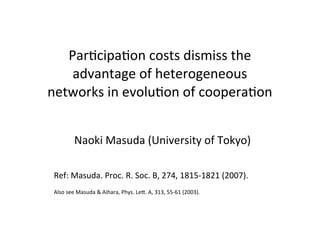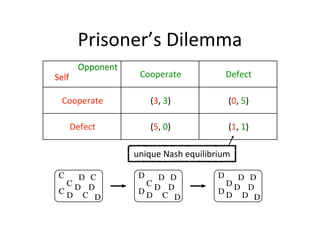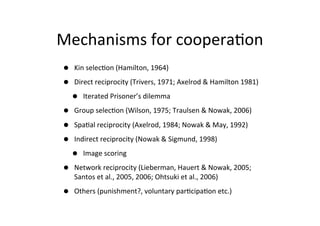Successfully reported this slideshow.

# Participation costs dismiss the advantage of heterogeneous networks in evolution of cooperation

0

Share×
1 of 26
1 of 26

# Participation costs dismiss the advantage of heterogeneous networks in evolution of cooperation

0

Share

Presentation slides for the following two papers (mainly (1)):
(1) Masuda. Proceedings of the Royal Society B: Biological Sciences, 274, 1815-1821 (2007).
(2) Masuda and Aihara. Physics Letters A, 313, 55-61 (2003).

Presentation slides for the following two papers (mainly (1)):
(1) Masuda. Proceedings of the Royal Society B: Biological Sciences, 274, 1815-1821 (2007).
(2) Masuda and Aihara. Physics Letters A, 313, 55-61 (2003).

## More Related Content

### Related Books

Free with a 14 day trial from Scribd

See all

### Related Audiobooks

Free with a 14 day trial from Scribd

See all

### Participation costs dismiss the advantage of heterogeneous networks in evolution of cooperation

1. 1. ParNcipaNon  costs  dismiss  the   advantage  of  heterogeneous   networks  in  evoluNon  of  cooperaNon Naoki  Masuda  (University  of  Tokyo) Ref:  Masuda.  Proc.  R.  Soc.  B,  274,  1815-­‐1821  (2007). Also  see  Masuda  &  Aihara,  Phys.  LeK.  A,  313,  55-­‐61  (2003).
2. 2. Prisoner’s  Dilemma Opponent Cooperate Defect Cooperate (3,  3) (0,  5) Defect (5,  0) (1,  1) Self unique  Nash  equilibrium C D C CD D CD C D D D D CD D DD C D D D D DD D DD D D
3. 3. Mechanisms  for  cooperaNon • • • • • • • Kin  selecNon  (Hamilton,  1964) Direct  reciprocity  (Trivers,  1971;  Axelrod  &  Hamilton  1981) • Iterated  Prisoner’s  dilemma Group  selecNon  (Wilson,  1975;  Traulsen  &  Nowak,  2006) SpaNal  reciprocity  (Axelrod,  1984;  Nowak  &  May,  1992) Indirect  reciprocity  (Nowak  &  Sigmund,  1998) • Image  scoring Network  reciprocity  (Lieberman,  Hauert  &  Nowak,  2005;   Santos  et  al.,  2005,  2006;  Ohtsuki  et  al.,  2006) Others  (punishment?,  voluntary  parNcipaNon  etc.)
4. 4. Iterated  Prisoner’s  Dilemma • Players  randomly  interact  with  others • Discount  factor  w  (0  ≤  w  ≤  1)  to  specify  the   prob.  that  the  next  game  is  played  in  a  round A  plays C D D C D C B  plays C C D C D D A  gets 3 5 1 3 1 0 A’s  accumulated  payoﬀ    =  3  +  5w  +  1w2  +  3w3  +  1w4  +  0w5  +  … acNon C D C (3,  3) (0,  5) D (5,  0) (1,  1)
5. 5. • SelecNon  based  on  accumulated  payoﬀ   aher  each  round • Replicator  dynamics • Best-­‐response  dynamics • Nice,  retalitatory,  and  forgiving  strategies   (e.g.  Tit-­‐for-­‐Tat)  are  generally  strong  (but   not  the  strongest).
6. 6. SpaNal  Prisoner’s  Dilemma (Axelrod,  1984;  Nowak  &  May,  1992) • e.g.  square  laice • Either  cooperator  or   C:24 C:18 D:24 D:12 defector  on  each   vertex • Each  player  plays   against  all  (4  or  8)   neighbors. C:24 C:21 C:12 D:16 C:24 C:21 C:12 D:16 C:21 C:15 D:20 D:16
7. 7. • • • Successful  strategies  propagate  aher  one  generaNon. Result:  Cs  form  “clusters”  to  resist  invasion  by  Ds. Note:  2-­‐neighbor  CA.  More  complex  than  1-­‐neighbor   dynamics  such  as  spin  (opinion)  dynamics  and  disease   dynamics
8. 8. PD  on  the  WaKs-­‐Strogatz  small-­‐world  network (Masuda  and  Aihara,  Phys.  LeK.  A,  2003) acNon C D C (1,  1) (0,  T) D (T,  0) (0,  0) p  =  0 p:  small p  ≈  1 1 0.8 0.6 %C 0.4 Note:  The  degrees  of  all  the   nodes  are  the  same   regardless  of  the  rewiring. 0.2 p=0 p=0.01 p=0.9 0 1 1.5 T 2 2.5
9. 9. large  p 1 •  1-­‐dim  ring •  QualitaNvely  the   0.8 0.6 %C 0.4 same  results  for  2-­‐dim   networks small  p 1 small  p 0.2 0 0 100 200 generation T=1.1 300 400 1 0.8 0.8 0.6 %C 0.4 0.6 %C 0.4 0.2 0.2 0 large  p 0 100 200 generation T=1.7 300 400 large  p 0 small  p T=3.0 0 100 200 generation 300 400
10. 10. more  C slow Clustering  helps  cooperaNon Small  distance  (L)  accelerates   whatever  propagaNon more  D fast
11. 11. Social  dilemma  games  on  scale-­‐free   networks?
12. 12. Scale-­‐free  networks  promote   cooperaNon acNon Cooperate Defect Cooperate (1,  1) (S,  T) Defect (T,  S) (0,  0) Regular  RG (or  the  complete  graph) 1 (a) no  dilemma snowdrih S 1 (b) S 0 0 stag  hunt -1 scale-­‐free 0 PD 1 T 2 -1 0 1 T 2 Originally  by  Santos,  Pacheco  &  Lenaerts,  PNAS  2006
13. 13. Two  assumpNons   underlie  the  enhanced   coopraNon  in  SF  nets
14. 14. AssumpNon  1:  addiNve  payoﬀ   scheme • AddiNve:  add  the  payoﬀs  gained  via  all  the   neighbors   • Nowak,  Bonhoeﬀer  &  May  1994;  Abramson  &   Kuperman  2001;  Ebel  &  Bornholdt,  2002;  Ihi,   Killingback  &  Doebeli  2004;  Durán  &  Mulet,  2005;   Santos  et  al.,  2005;  2006;  Ohtsuki  et  al.,  2006 • Average:  divide  the  summed  payoﬀs  by  the  number   of  neighbors • Kim  et  al.,  2002;  Holme  et  al.,  2003;  Vukov  &   Szabó,  2005;  Taylor  &  Nowak,  2006
15. 15. acNon Cooperate Defect Cooperate (3,  3) (0,  5) Defect (5,  0) (1,  1) 10 90 v1 k 1 = 100 v2 k2 = 2 =C =D payoﬀ  scheme addiNve 3×10+0×90  =  30 5×2  =  10 average (3×10+0×90)/100  =  0.3 (5×2)/2  =  5
16. 16. • Average  payoﬀ  diminishes  cooperaNon  in   heterogeneous  networks  (Santos  &   Pacheco,  J.  Evol.  Biol.,  2006).
17. 17. AssumpNon  2:  PosiNvely  biased   payoﬀs • C D C a b D c d ( Originate  from  the  translaNon  invariance  of  replicator  dynamics ! ! ( ⇡C = ⇡D = axC + bxD cxC + dxD h⇡i = ⇡C xC + ⇡D xD xC = ˙ xD = ˙ xC (⇡C xD (⇡D ✓ a b c d ◆ ✓ ◆ h b h →  parNcipaNon   h d h cost ✓ ◆ ✓ ◆ a b ka kb ! →  Nme  rescaling c d kc kd ◆ ◆ ✓ ✓ a b a+k b+k ! c d c d ! a c h⇡i) h⇡i) acNon C D acNon C D C (1,  1) (0,  T) C (1,  1) (S,  T) D (T,  0) (0,  0) D (T,  S) (0,  0)
18. 18. Reason  for  enhanced  cooperaNon • Hubs  earn  more  than   leaves. • C  on  hubs  (with  at  least   some  C  neighbors)  are   stable. • C  spreads  from  hubs  to   leaves. 10 acNon C D C (1,  1) (0,  T) D (T,  0) (0,  0) 90 v1 k 1 = 100 v2 k2 = 2 =C =D
19. 19. Payoﬀ  matrix  is  not  invariant  on   heterogeneous  networks C D C a b D c d ( ! ! ( ⇡C = ⇡D = ✓ h⇡i = ⇡C xC + ⇡D xD xC = ˙ xD = ˙ xC (⇡C xD (⇡D ✓ ! h⇡i) h⇡i) acNon C D C (1,  1) (0,  T) D (T,  0) (0,  0) a c ◆ h b h →  parNcipaNon   h d NG h cost ✓ ◆ ✓ ◆ a b ka kb ! ✔ →  Nme  rescaling c d kc kd ◆ ◆ ✓ ✓ a b a+k b+k ! NG c d c d axC + bxD cxC + dxD a b c d ◆
20. 20. Our  assumpNons • AddiNve  payoﬀ  scheme • Introduce  the  parNcipaNon  cost • Do  numerics • N  =  5000  players • Each  player  plays  against  all  the  neighbors. • Replicator-­‐type  update  rule:  player  i  copies   player  j’s  strategy  with  prob  (πj-­‐πi)/[max(ki,kj)  *  (max  possible   payoﬀ  –  min  possible  payoﬀ)]
21. 21. Simpliﬁed  prisoner’s  dilemma regular  random  net (a) 3 1 cf 0.75 2 0.5 1 0.25 0 acNon C D C (1-h,  1-h) (-h,  T-h) D (T-h,  -h) 0 h 0.7 1 T 1.3 scale-­‐free  net 1.6 (-h,  -h) 3  (roughly  separated)  regimes Strong  inﬂuence  of  iniNal  cnds  due   1 to  long  transients (a) 3 2 Somewhat  reduced  cooperaNon h 1 2 Enhanced  cooperaNon  (prev  results) 3 0 0.7 1 T 1.3 1.6
22. 22. T  =  1.5 (b) h  =  0 100 50 h  =  0.2 h  =  0.23 0 h  =  0.24 h  =  0.25 -50 h  =  0.5 0 50 h  =  0.3 100 150 # neighbors 200 h  =  0.2 h  =  0 # flips generation payoff (a) h  =  0.5 50 h  =  0.23 h  =  0.3 h  =  0.24 h  =  0.25 0 10 100 # neighbors Strategy  spreads  from  stubborn   leaves  to  hubs. h 1 0 0.7 1 T 1.3 1.6 2 From  hub  cooperators  to  leaves. 2 1 From  leaves  to  hubs.    PD  payoﬀ   structure  is  most  relevant. (a) 3 3
23. 23. General  matrix  game • Homogeneous  (in  degree)  →  2  parameters  (S   and  T) • e.g.  well-­‐mixed,  square  laice,  regular   random  graph • Heterogeneous  →  3  parameters  (S,  T,  and  h) • e.g.  ER  random  graph,  scale-­‐free • PD,  snowdrih  game,  hawk-­‐dove  game  included acNon C D C (1-h,  1-h) (S-h,  T-h) D (T-h,  S-h) (-h,  -h)
24. 24. consistent  with  Santos  et  al.,  PNAS  (2006) Regular  RG  (h  =  0) 1 (a) SF  (h  =  0.5) snowdrih 0 1 (b) 1 (c) S no  dilemma S S 0 stag  hunt -1 SF  (h  =  0) 0 PD 1 T 1 2 -1 0 1 0 SF  (h  =  1) 1 T 2 SF  (h  =  2) 1 (d) -1 2 0 1 1 cf 1 (e) 0.75 S S 0 -1 0.5 0 2 0 1 T 2 -1 3 0 1 T 2 0.25 0 T 2
25. 25. Thoughts  about  the  payoﬀ  bias   • Naturally  understood  as  the  parNcipaNon  cost • Payoﬀs  may  be  negaNve  in  many  pracNcal   situaNons. • Environmental  problems? • InternaNonal  relaNons? • When  one  is  ‘forced’  to  play  games
26. 26. Conclusions • Games  with  parNcipaNon  costs  on  networks   • More  C  for  small  parNcipaNon  cost  h   (previous  work). • Networks  determine  dynamics  for  small  and   large  h. • Payoﬀ  matrix  is  most  relevant  for   intermediate  h. • Think  twice  about  the  use  of  simpliﬁed  PD   payoﬀ  matrices.